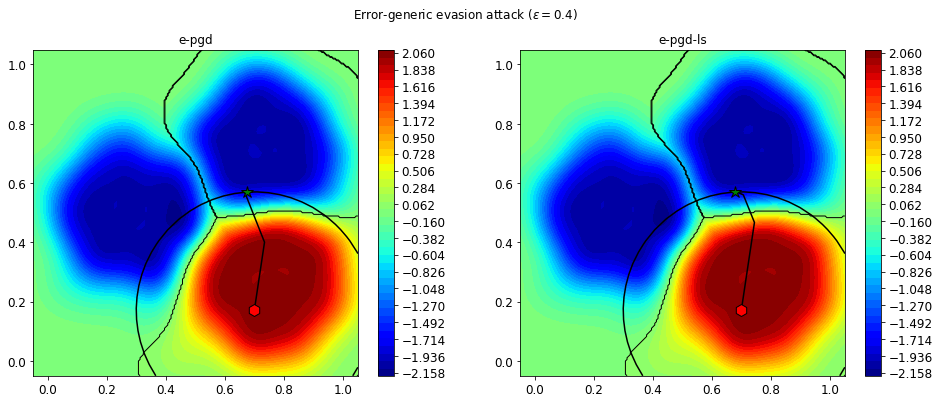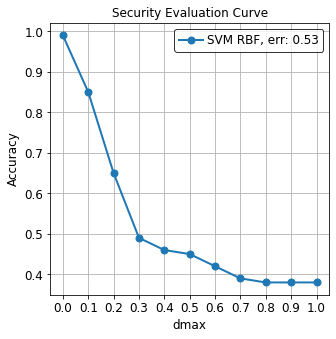# 2.1. Evasion Attacks against Machine Learning¶

In this tutorial we will experiment with adversarial evasion attacks against a Support Vector Machine (SVM) with the Radial Basis Function (RBF) kernel.

Evasion attacks (a.k.a. adversarial examples) consists of carefully perturbing the input samples at test time to have them misclassified.

We will first create and train the classifier, evaluating its performance in the standard scenario, i.e. not under attack.

The following part replicates the procedure from the first tutorial.:

%%capture --no-stderr --no-display
# NBVAL_IGNORE_OUTPUT

try:
import secml
except ImportError:
%pip install git+https://gitlab.com/secml/secml

:

random_state = 999

n_features = 2  # Number of features
n_samples = 1100  # Number of samples
centers = [[-2, 0], [2, -2], [2, 2]]  # Centers of the clusters
cluster_std = 0.8  # Standard deviation of the clusters

dataset = CDLRandomBlobs(n_features=n_features,
centers=centers,
cluster_std=cluster_std,
n_samples=n_samples,

n_tr = 1000  # Number of training set samples
n_ts = 100  # Number of test set samples

# Split in training and test
from secml.data.splitter import CTrainTestSplit
splitter = CTrainTestSplit(
train_size=n_tr, test_size=n_ts, random_state=random_state)
tr, ts = splitter.split(dataset)

# Normalize the data
from secml.ml.features import CNormalizerMinMax
nmz = CNormalizerMinMax()
tr.X = nmz.fit_transform(tr.X)
ts.X = nmz.transform(ts.X)

# Metric to use for training and performance evaluation
from secml.ml.peval.metrics import CMetricAccuracy
metric = CMetricAccuracy()

# Creation of the multiclass classifier
from secml.ml.classifiers import CClassifierSVM
from secml.ml.classifiers.multiclass import CClassifierMulticlassOVA
from secml.ml.kernels import CKernelRBF
clf = CClassifierMulticlassOVA(CClassifierSVM, kernel=CKernelRBF())

# Parameters for the Cross-Validation procedure
xval_params = {'C': [1e-2, 0.1, 1], 'kernel.gamma': [10, 100, 1e3]}

# Let's create a 3-Fold data splitter
from secml.data.splitter import CDataSplitterKFold
xval_splitter = CDataSplitterKFold(num_folds=3, random_state=random_state)

# Select and set the best training parameters for the classifier
print("Estimating the best training parameters...")
best_params = clf.estimate_parameters(
dataset=tr,
parameters=xval_params,
splitter=xval_splitter,
metric='accuracy',
perf_evaluator='xval'
)

print("The best training parameters are: ",
[(k, best_params[k]) for k in sorted(best_params)])

# We can now fit the classifier
clf.fit(tr.X, tr.Y)

# Compute predictions on a test set
y_pred = clf.predict(ts.X)

# Evaluate the accuracy of the classifier
acc = metric.performance_score(y_true=ts.Y, y_pred=y_pred)

print("Accuracy on test set: {:.2%}".format(acc))

Estimating the best training parameters...
The best training parameters are:  [('C', 0.1), ('kernel.gamma', 100)]
Accuracy on test set: 99.00%


We are going to create an adversarial example against the SVM classifier using the gradient-based maximum-confidence algorithm for generating evasion attacks proposed in:

[biggio13-ecml] Biggio, B., Corona, I., Maiorca, D., Nelson, B., Šrndić, N., Laskov, P., Giacinto, G., Roli, F., 2013. Evasion Attacks against Machine Learning at Test Time. In ECML-PKDD 2013.

[melis17-vipar] Melis, M., Demontis, A., Biggio, B., Brown, G., Fumera, G. and Roli, F., 2017. Is deep learning safe for robot vision? adversarial examples against the icub humanoid. In Proceedings of IEEE ICCV 2017.

[demontis19-usenix] Demontis, A., Melis, M., Pintor, M., Jagielski, M., Biggio, B., Oprea, A., Nita-Rotaru, C. and Roli, F., 2019. Why Do Adversarial Attacks Transfer? Explaining Transferability of Evasion and Poisoning Attacks. In 28th Usenix Security Symposium, Santa Clara, California, USA.

which is implemented in SecML by the CAttackEvasionPGDLS class.

Let’s define the attack parameters. First, we choose to generate an l2 perturbation within a maximum ball of radius eps = 0.4 from the initial point. The maximum perturbation value is denoted as dmax in our implementation. Second, we also add a low/upper bound as our feature space is limited in [0, 1]. Last, as we are not interested in generating an adversarial example for a specific class, we perform an error-generic attack by setting y_target = None.

The attack internally uses a solver based on Projected Gradient Descent with Bisect Line Search, implemented by the COptimizerPGDLS class. The parameters of the solver can be specified while creating the attack and must be optimized depending on the specific optimization problem.

:

x0, y0 = ts[5, :].X, ts[5, :].Y  # Initial sample

noise_type = 'l2'  # Type of perturbation 'l1' or 'l2'
dmax = 0.4  # Maximum perturbation
lb, ub = 0, 1  # Bounds of the attack space. Can be set to None for unbounded
y_target = None  # None if error-generic or a class label for error-specific

# Should be chosen depending on the optimization problem
solver_params = {
'eta': 0.3,
'eta_min': 0.1,
'eta_max': None,
'max_iter': 100,
'eps': 1e-4
}

pgd_ls_attack = CAttackEvasionPGDLS(
classifier=clf,
double_init_ds=tr,
double_init=False,
distance=noise_type,
dmax=dmax,
lb=lb, ub=ub,
solver_params=solver_params,
y_target=y_target)

# Run the evasion attack on x0
y_pred_pgdls, _, adv_ds_pgdls, _ = pgd_ls_attack.run(x0, y0)

print("Original x0 label: ", y0.item())
print("Adversarial example label (PGD-LS): ", y_pred_pgdls.item())

print("Number of classifier gradient evaluations: {:}"

Original x0 label:  1
Number of classifier gradient evaluations: 7


Let’s now test another attack algorithm, implemented by CAttackEvasionPGD, which leverages the standard Projected Gradient Descent solver. We keep the same attack parameters as before.

:

# Should be chosen depending on the optimization problem
solver_params = {
'eta': 0.3,
'max_iter': 100,
'eps': 1e-4
}

pgd_attack = CAttackEvasionPGD(
classifier=clf,
double_init_ds=tr,
double_init=False,
distance=noise_type,
dmax=dmax,
lb=lb, ub=ub,
solver_params=solver_params,
y_target=y_target)

# Run the evasion attack on x0
y_pred_pgd, _, adv_ds_pgd, _ = pgd_attack.run(x0, y0)

print("Original x0 label: ", y0.item())
print("Adversarial example label (PGD): ", y_pred_pgd.item())

print("Number of classifier gradient evaluations: {:}"


Original x0 label:  1
Number of classifier gradient evaluations: 40


We can see that the classifier has been successfully evaded in both cases. However, the PGD-LS solver with bisect line search queries the classifier gradient function many times less, thus generating the adversarial examples much faster.

Let’s now visualize both the attacks on a 2D plane. On the background, the value of the objective function of the attacks is shown.

:

from secml.figure import CFigure
# Only required for visualization in notebooks
%matplotlib inline

fig = CFigure(width=16, height=6, markersize=12)

# Let's replicate the l2 constraint used by the attack for visualization
from secml.optim.constraints import CConstraintL2

for i, (attack, adv_ds) in enumerate(

fig.subplot(1, 2, i + 1)

# Convenience function for plotting the attack objective function
fig.sp.plot_fun(attack.objective_function, plot_levels=False,
multipoint=True, n_grid_points=200)
# Let's also plot the decision boundaries of the classifier
fig.sp.plot_decision_regions(clf, plot_background=False, n_grid_points=200)

# Construct an array with the original point and the adversarial example

# Convenience function for plotting the optimization sequence
fig.sp.plot_path(attack.x_seq)

# Convenience function for plotting a constraint
fig.sp.plot_constraint(constraint)

fig.sp.title(attack.class_type)
fig.sp.grid(grid_on=False)

fig.title(r"Error-generic evasion attack ($\varepsilon={:}$)".format(dmax))

fig.show()We can see that the initial point x0 (red hexagon) has been perturbed in the feature space so that is actually classified by the SVM as a point from another class. The final adversarial example is the green star. We also show the l2 constraint as a black circle which has limited the maximum perturbation applicable to x0.

## 2.1.2. Security evaluation of a classifier¶

We could be interested in evaluating the robustness of a classifier against increasing values of the maximum perturbation eps.

SecML provides a way to easily produce a Security Evaluation Curve, by means of the CSecEval class.

The CSecEval instance will take a CAttack as input and will test the classifier using the desired perturbation levels.

Please note that the security evaluation process may take a while (up to a few minutes) depending on the machine the script is run on.

:

# Perturbation levels to test
from secml.array import CArray
e_vals = CArray.arange(start=0, step=0.1, stop=1.1)

sec_eval = CSecEval(
attack=pgd_ls_attack, param_name='dmax', param_values=e_vals)

# Run the security evaluation using the test set
print("Running security evaluation...")
sec_eval.run_sec_eval(ts)

from secml.figure import CFigure
fig = CFigure(height=5, width=5)

# Convenience function for plotting the Security Evaluation Curve
fig.sp.plot_sec_eval(
sec_eval.sec_eval_data, marker='o', label='SVM RBF', show_average=True)

Running security evaluation...We can see how the SVM classifier is vulnerable to adversarial attacks and we are able to evade it even with small perturbations.

For further reference about the security evaluation of machine-learning models under attack see:

[biggio13-tkde] Biggio, B., Fumera, G. and Roli, F., 2013. Security evaluation of pattern classifiers under attack. In IEEE transactions on knowledge and data engineering.

[biggio18-pr] Biggio, B. and Roli, F., 2018. Wild patterns: Ten years after the rise of adversarial machine learning. In Pattern Recognition.